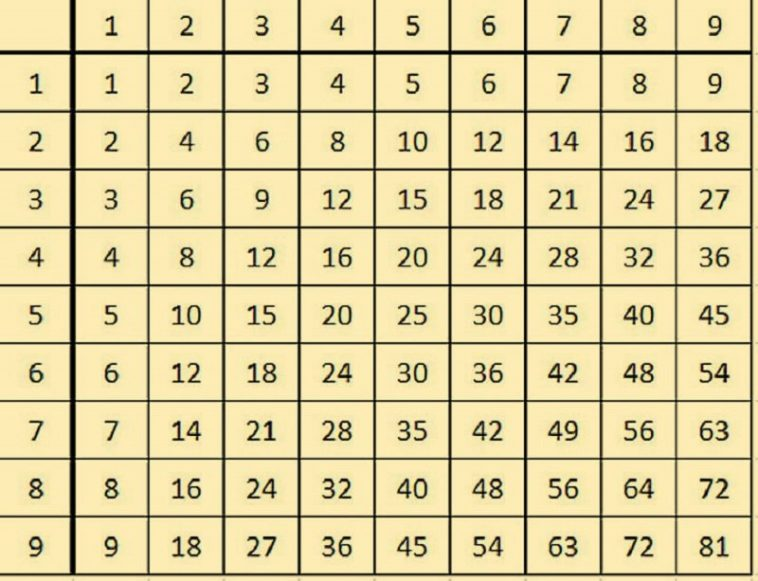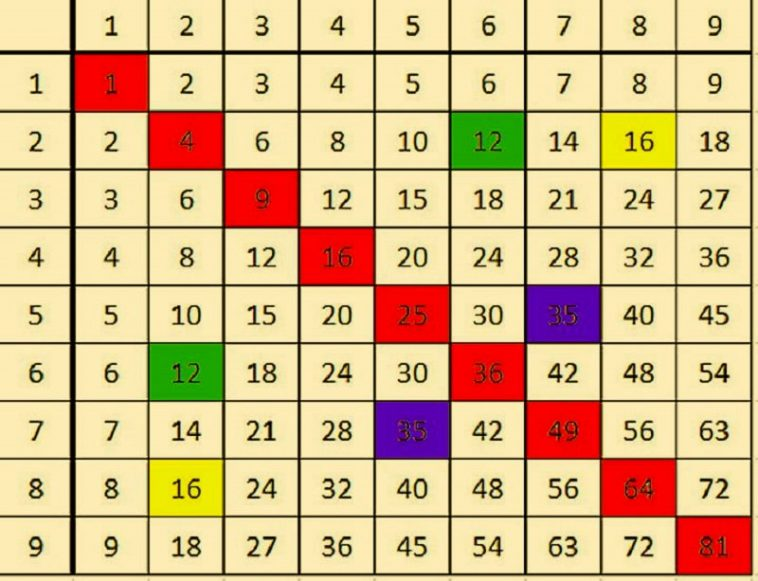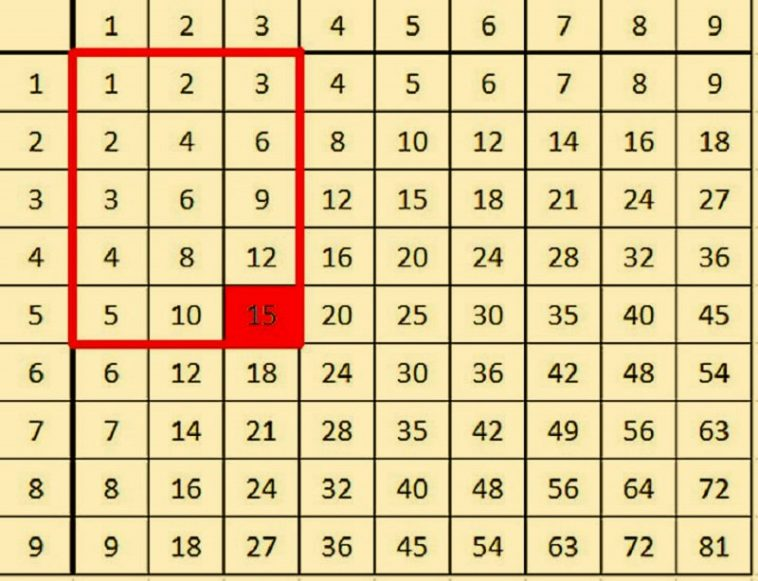# This Table Can Teach a Child or Adult to Multiply Quickly And Effectively!

Learning is one of the most important activities for any human being regardless of age. Children can learn things easily and quickly thanks to their developing brain and mental agility, but things are far slower in older people. As we age, our ability to learn is significantly reduced and even multiplying simple numbers becomes hard. This activity is simple when we’re young – children can memorize multiplication tables one by one, but there is an easier way of learning how to multiply without much effort.

The best method for learning how to multiply numbers is the Pythagoras table. This table will make things easier for you and will help you learn how to multiply through observation and logic. Maybe some of you have heard about it – if you know the Pythagoras table, you know how helpful it is. If you don’t know it, here’s a chance of helping your children learn how to multiply fast.

Pythagoras table

The Pythagorean table is made up of 9 rows and columns of numbers that go from 1 to 10. The point which intersects a row with a certain column is a result of multiplying the numbers on the edges. Here’s a simple example that will help you understand – if you want to multiply 7 by 2, you need to get to row 7 in the first column and move to the right until you reach the column with the number 2. The point where these two numbers meet is the multiplication result (in this case 14).The table offers much more benefits that we’ll present later. For now, we want to show you that it’s fairly easy to learn how to multiply with it. If you learn how to use it, multiplying numbers will be a breeze – just understand the concept of the table and you’re good to go. Once you make yourself comfortable with it, you can move on to complex multiplication.

Follow the red diagonal fields in the picture – they represent the center of the table. Now, look at the numbers just below the diagonal fields. They highlighted numbers are there to improve the observation and logical reasoning of your children and help them find patters in the table.Now, the next image shows how the symmetry of the table comes into play. If you multiply 5×3 you’ll get 15, but if you look more closely, you’ll see that it forms a rectangle with the same amount of cells.This is just one of the advantages the Pythagoras table offers. If you make your children learn how to use it, they will see that learning how to multiply is easy and fun. This will make them get better grades at school and become better at reasoning and logic. Start using the table today and you will be amazed by the results!